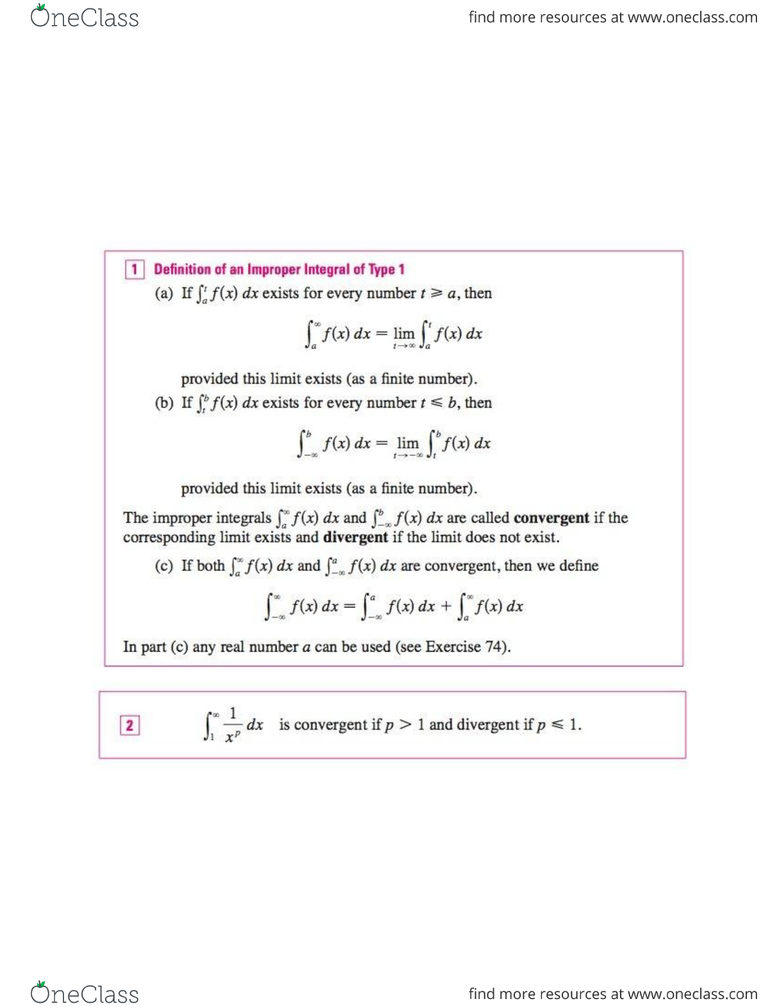Textbook Notes (280,000)
US (110,000)
UCSB (700)
MATH 3B (10)
helms (10)
Chapter 7.8

# MATH 3B Chapter Notes - Chapter 7.8: Improper Integral, Asymptote

Page:
of 2Chapter 7.8 Improper Integrals
improper integral: concept of a definite integral from a to b f(x) dx, we dealt with a function f
defined on a finite interval [a, b] and we assumed that f does not have an infinite discontinuity or
definite integral to the case where the interval is infinite and also to the case where f has an
infinite discontinuity in [a,b]
Type 1: Infinite Intervals
Type 2: Discontinuous Integrands
WARNING If we had not noticed the asymptote, we might have made an erroneous calculation.
This is wrong because the integral is improper and must be calculated in terms of limits.
A Comparison Test for Improper Integrals